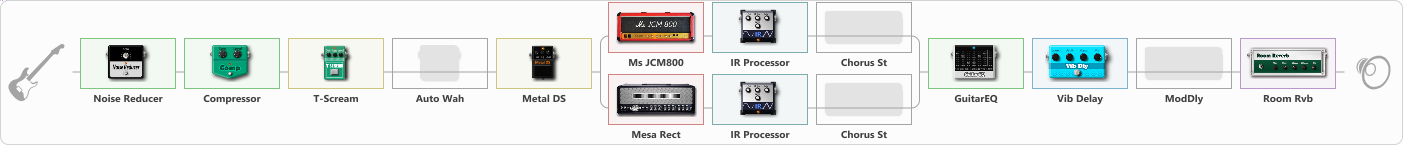# JCM 800 + MESA

Discussion in 'ToneLib-GFX presets' started by Summers, Jul 8, 2023.

1. JCM 800 + MESA

Preset name: just another mesa

HEAVY

Effects chain:Effect: "Noise Reducer" (Dynamics / Filter), active - "yes"
{
"Sens" = 66
"Mode" = Hard
}

Effect: "Compressor" (Dynamics / Filter), active - "yes"
{
"Sense" = 34
"Level" = 50
}

Effect: "T-Scream" (Overdrive / Distortion), active - "yes"
{
"Drive" = 0
"Tone" = 100
"Level" = 100
}

Effect: "Auto Wah" (Dynamics / Filter), active - "no"
{
"Sense" = 55
"Polarity" = Up
"Attack" = 35
"Style" = V848
}

Effect: "Metal DS" (Overdrive / Distortion), active - "yes"
{
"Dist" = 47
"Bass" = 56
"Middle" = 60
"Treble" = 46
"Level" = 57
}

Effect: "Splitter" (Dynamics / Filter)
{
"A-Bypass" = Off
"A-Pan" = -25
"A-Level" = 51
"B-Bypass" = Off
"B-Pan" = 25
"B-Level" = 50
"Width" = 0

'A' branch:
{

Effect: "Ms JCM800" (Amp simulators), active - "yes"
{
"Gain" = 81
"Bass" = 48
"Middle" = 69
"Treble" = 70
"Presence" = 67
"Master" = 69
"Level (dB)" = 4
}

Effect: "IR Processor" (Cabinets), active - "yes"
{
"IR" = Mesa 4x12 SPKR 1 position 2
"Low Cut (Hz)" = 45
"Hi Cut (kHz)" = 20.0
"Mix" = 100
"Level (dB)" = 0
}

Effect: "Chorus St" (Modulation / Sfx), active - "no"
{
"Speed" = 1.9
"Depth" = 31
"Center" = 4.4
"Mix" = 29
}
}
'B' branch:
{

Effect: "Mesa Rect" (Amp simulators), active - "yes"
{
"Gain" = 66
"Bass" = 38
"Middle" = 0
"Treble" = 65
"Presence" = 70
"Master" = 77
"Level (dB)" = 4
}

Effect: "IR Processor" (Cabinets), active - "yes"
{
"IR" = Mesa 4x12 SPKR 1 position 2
"Low Cut (Hz)" = 54
"Hi Cut (kHz)" = 20.0
"Mix" = 100
"Level (dB)" = 0
}

Effect: "Chorus St" (Modulation / Sfx), active - "no"
{
"Speed" = 1.9
"Depth" = 31
"Center" = 4.4
"Mix" = 29
}
}
}

Effect: "GuitarEQ" (Dynamics / Filter), active - "yes"
{
"160 Hz" = -1
"400 Hz" = -1
"800 Hz" = 2
"1.6 kHz" = 1
"3.2 kHz" = 2
"6.4 kHz" = -1
"12 kHz" = 0
"Level (dB)" = 0
}

Effect: "Vib Delay" (Modulation / Sfx), active - "yes"
{
"Speed" = 4.2
"Depth" = 16
"Feedback" = 27
"Time" = 5.7
"Mix" = 36
"Mode" = 0
}

Effect: "ModDly" (Delay), active - "no"
{
"Time" = 375
"Feedback" = 52
"Tone" = 70
"Speed" = 1.9
"Mix" = 44
}

Effect: "Room Rvb" (Reverberation), active - "yes"
{
"Time" = 4.5
"PreDelay" = 0
"LoDamp" = 46
"HiDamp" = 25
"Mix" = 35
}

Note: You will need to download and install the ToneLib-GFX software to use the preset.

File size:
58.1 KB
Views:
1,724